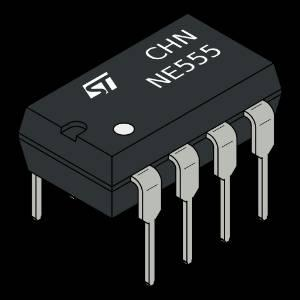Light Sensitive Sampling Process Microcontroller Software and Hardware Application (MSHA) Project.

IntermediateFull instructions provided111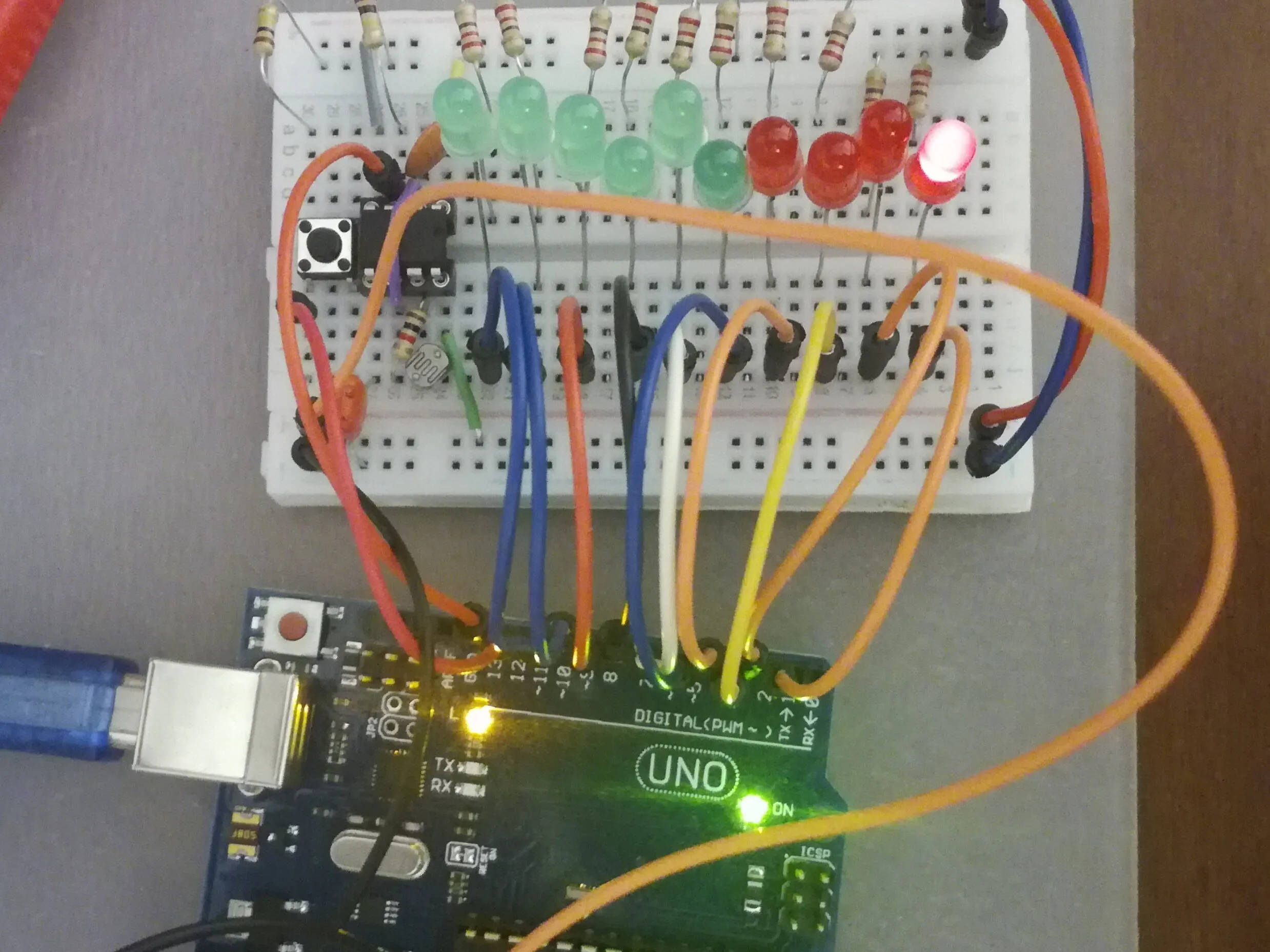## Things used in this project

### Hardware componentsArduino UNO
×1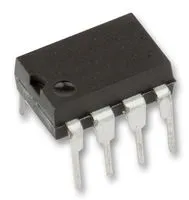555 Timers
×1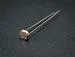Photo resistor
×1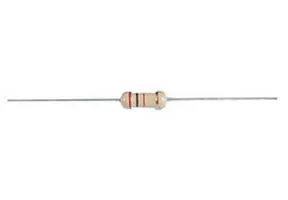Through Hole Resistor, 680 ohm
×1
 Ceramic Capacitor 1.2 nF
×1
 Ceramic Capacitor 10 nF
×1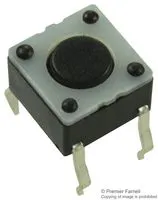Tactile Switch, Top Actuated
×1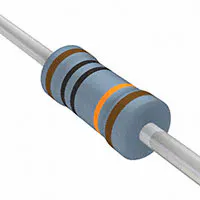Resistor 100k ohm
×2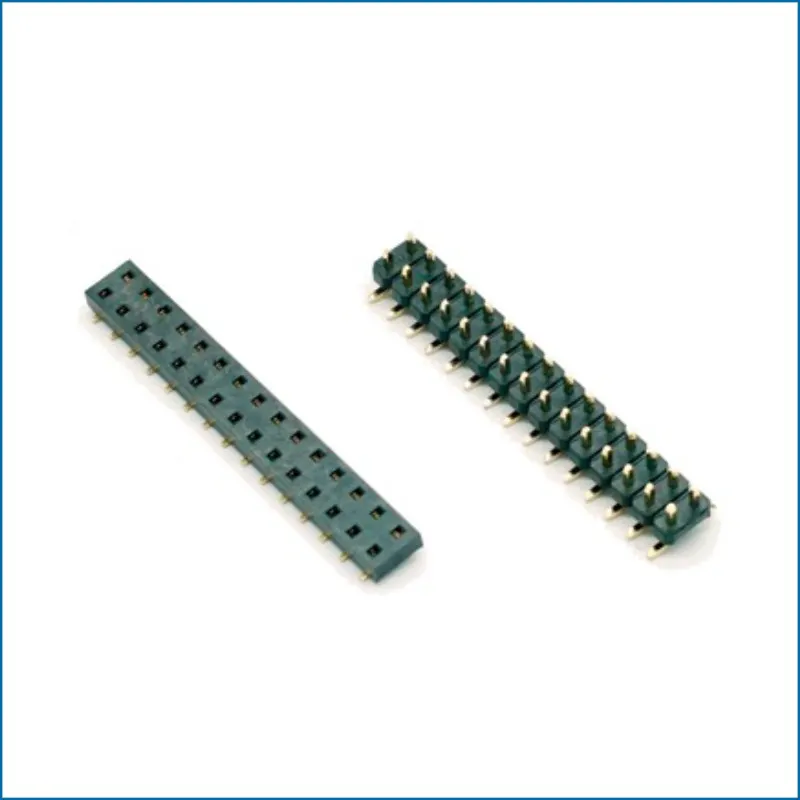M5Stack 2x15 Pin Headers Socket 2.54mm Male & Female 4 Pair Connector
×14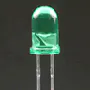5 mm LED: Green
×10Resistor 220 ohm
×10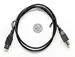USB-A to B Cable (If need to connect to PC or 5V powerbank)
×1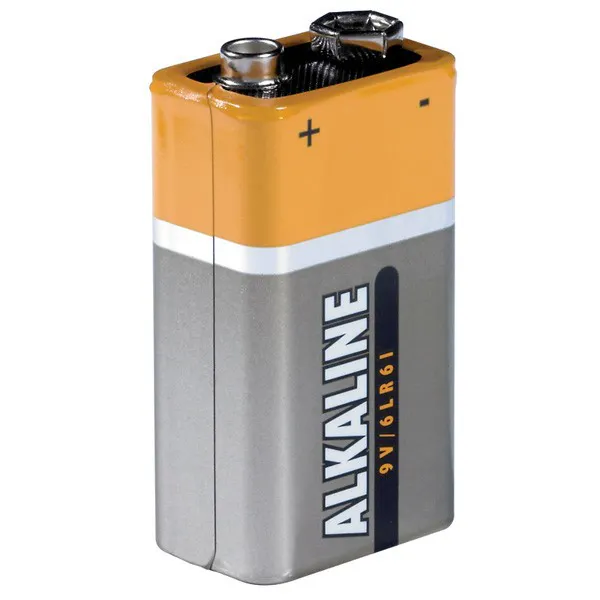9V battery (generic) (If not connected to PC or 5V powerbank)
×1
 Male/Male Jumper Wires
×1

### Hand tools and fabrication machinesSoldering iron (generic)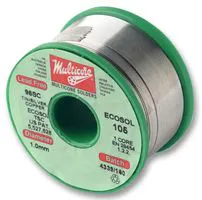## Schematics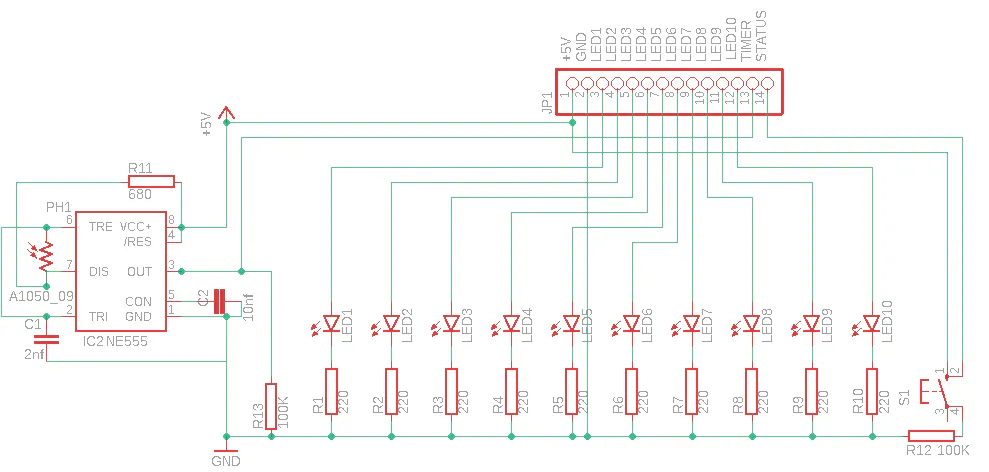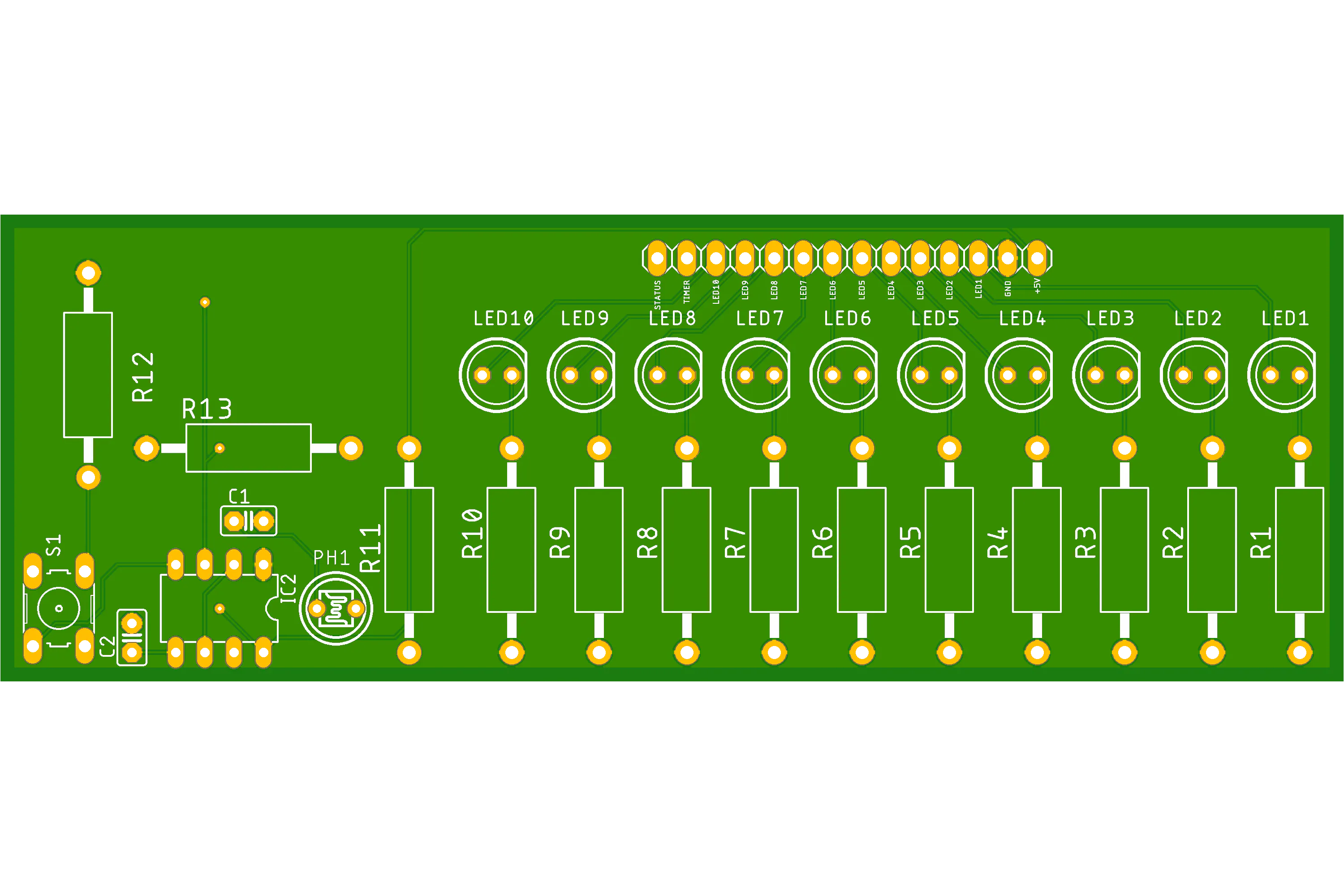## Code

Arduino
```int statesMaxLength = 600;

class Timer555
{
public:
int pin;
Timer555(int pin)
{
this->pin = pin;
}
int getCurrentState()
{
}
};

class LiteScopeBoard
{
private:
unsigned long int millisPerHour = 3600000;
float T = 834.00;
float T_59 = this->T * 59.00 / 100.00;
float T_41 = this->T * 41.00 / 100.00;
float T_ratio = this->T_59 / this->T_41;
float float_stm = float(statesMaxLength);
float boardClock = 16000.00;
float clock_ratio = this->boardClock / this->float_stm;
float num_of_ones = 0.00;
float num_of_zeros = 0.00;
float one_cycle = 1.00;
int ledNum = 10;
int ledPins;
int timerPin;
int switchModePin;
int getTimerPin(Timer555 t)
{
return t.pin;
}

public:
unsigned long int counter = 0;
float cycles = 0.00;
LiteScopeBoard(Timer555 timer, int switchModePin, int l0, int l1, int l2, int l3, int l4, int l5, int l6, int l7, int l8, int l9)
{
this->timerPin = this->getTimerPin(timer);
this->switchModePin = switchModePin;
for (int i = 0; i < this->ledNum; i++)
{
switch (i)
{
case 0:
this->ledPins[i] = l0;
break;
case 1:
this->ledPins[i] = l1;
break;
case 2:
this->ledPins[i] = l2;
break;
case 3:
this->ledPins[i] = l3;
break;
case 4:
this->ledPins[i] = l4;
break;
case 5:
this->ledPins[i] = l5;
break;
case 6:
this->ledPins[i] = l6;
break;
case 7:
this->ledPins[i] = l7;
break;
case 8:
this->ledPins[i] = l8;
break;
case 9:
this->ledPins[i] = l9;
break;
}
}
}
void timerPinSetup()
{
pinMode(this->timerPin, INPUT);
}
void switchModePinSetup()
{
pinMode(this->switchModePin, INPUT);
}
void ledPinsSetup()
{
for (int i = 0; i < this->ledNum; i++)
{
pinMode(this->ledPins[i], OUTPUT);
}
}
void initialize()
{
delay(1000);
this->allLedsON();
delay(1000);
this->allLedsOFF();
if (Serial)
{
Serial.print("Timer Pin: ");
Serial.println(this->timerPin);
Serial.print("Switch Mode Pin: ");
Serial.println(this->switchModePin);
Serial.println();
}
// sync / snap board to a timer's first and nearest ON state (logical 1)
{
;
}
}
int getMode()
{
}
void count()
{
this->counter++;
}
{
int state, del;
float cyclesRatio, runCycles;
for (int j = 0; j < 2; j++)
{
for (int i = 0; i < statesMaxLength; i++)
{
state = t.getCurrentState();
switch (state)
{
case 0:
this->num_of_zeros++;
break;
case 1:
this->num_of_ones++;
break;
}
}
}
if (this->num_of_zeros != 0 && this->num_of_ones != 0)
{
cyclesRatio = float(this->num_of_ones) / float(this->num_of_zeros);
cyclesRatio /= this->T_ratio;
runCycles = this->one_cycle / cyclesRatio;
runCycles *= this->clock_ratio;
this->cycles += runCycles;
}
this->num_of_zeros = 0.00;
this->num_of_ones = 0.00;
del = int(this->T);
delayMicroseconds(del);
}
void ledON(int i)
{
digitalWrite(this->ledPins[i], HIGH);
}
void ledOFF(int i)
{
digitalWrite(this->ledPins[i], LOW);
}
void allLedsON()
{
for (int i = 0; i < this->ledNum; i++)
{
this->ledON(i);
}
}
void allLedsOFF()
{
for (int i = 0; i < this->ledNum; i++)
{
this->ledOFF(i);
}
}
void showStatus()
{
if (this->getMode() == 1)
{
if (Serial)
{
Serial.print("Board Loops counted: ");
Serial.print(this->counter);
Serial.println();
Serial.print("Timer Cycles counted: ");
Serial.print(this->cycles);
Serial.println();
Serial.println();
}
}
}
{
float float_hr;
int int_hr;
float_hr = this->cycles / float(this->millisPerHour);
int_hr = int(float_hr);
{
if (int_hr >= 1 && int_hr <= 10)
{
}
}
}
{
{
for (int i = 0; i < this->Helliogrades; i++)
{
this->ledON(i);
}
}
}
};

Timer555 timer(13);
LiteScopeBoard board(timer, 12, 2, 3, 4, 5, 6, 7, 8, 9, 10, 11);

void setup()
{
// **  THE FOLLOWING CODE WORKS PERFECT ON ARDUINO.  **
// **  SO, WE CAN INITIALIZE LOOP, AFTER SYNCING
//     BOARD TO TIMER AT THE VERY FIRST TIMER'S
//     ON STATE MOMENT
/*Serial.begin(9600);
pinMode(2, INPUT);
delay(1000);
;
}
Serial.println("ok");*/
Serial.begin(115200);
board.timerPinSetup();
board.switchModePinSetup();
board.ledPinsSetup();
board.initialize();
}

void loop()
{
board.count();